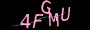×

# 详细了解下跟数学相关的JavaScript之Math对象Math对象包含许多与数学相关的实用程序。我们在这里看看都有哪些。

```var pi_value=Math.PI;
var sqrt_value=Math.sqrt(15);```

### 常量

`Math.E`常数e，自然对数的基数（表示~2.71828）
`Math.LN10`表示 10 的自然对数，约为 2.302
`Math.LN2`表示 2 的自然对数，约为 0.693
`Math.LOG10E`表示以 10 为底数，e 的对数，约为 0.434
`Math.LOG2E`表示以 2 为底数，e 的对数，约为 1.442
`Math.PI`表示一个圆的周长与直径的比例，约为 3.14159
`Math.SQRT1_2`表示 1/2 的平方根，约为 0.707
`Math.SQRT2`表示 2 的平方根，约为 1.414

### 函数

#### Math.abs()

JavaScript 代码:

```Math.abs(2.5) //2.5
Math.abs(-2.5) //2.5```

#### Math.acos()

JavaScript 代码:

`Math.acos(0.8) //0.6435011087932843`

#### Math.asin()

JavaScript 代码:

`Math.asin(0.8) //0.9272952180016123`

#### Math.atan()

JavaScript 代码:

`Math.atan(30) //1.5374753309166493`

#### Math.atan2()

JavaScript 代码:

`Math.atan2(30, 20) //0.982793723247329`

#### Math.ceil()

JavaScript 代码:

```Math.ceil(2.5) //3
Math.ceil(2) //2
Math.ceil(2.1) //3
Math.ceil(2.99999) //3```

#### Math.cos()

JavaScript 代码:

```Math.cos(0) //1
Math.cos(Math.PI) //-1```

#### Math.exp()

JavaScript 代码:

```Math.exp(1) //2.718281828459045
Math.exp(2) //7.38905609893065
Math.exp(5) //148.4131591025766```

#### Math.floor()

JavaScript 代码:

```Math.ceil(2.5) //2
Math.ceil(2) //2
Math.ceil(2.1) //2
Math.ceil(2.99999) //2```

#### Math.log()

JavaScript 代码:

```Math.log(10) //2.302585092994046
Math.log(Math.E) //1```

#### Math.max()

JavaScript 代码:

```Math.max(1,2,3,4,5) //5
Math.max(1) //1```

#### Math.min()

JavaScript 代码:

```Math.min(1,2,3,4,5) //1
Math.min(1) //1```

#### Math.pow()

JavaScript 代码:

```Math.pow(1, 2) //1
Math.pow(2, 1) //2
Math.pow(2, 2) //4
Math.pow(2, 4) //16```

#### Math.random()

JavaScript 代码:

```Math.random() //0.9318168241227056
Math.random() //0.35268950194094395```

#### Math.round()

JavaScript 代码:

```Math.round(1.2) //1
Math.round(1.6) //2```

#### Math.sin()

JavaScript 代码:

```Math.sin(0) //0
Math.sin(Math.PI) //1.2246467991473532e-16)```

#### Math.sqrt()

JavaScript 代码:

```Math.sqrt(4) //2
Math.sqrt(16) //4
Math.sqrt(5) //2.23606797749979```

#### Math.tan()

JavaScript 代码:

```Math.tan(0) //0
Math.tan(Math.PI) //-1.2246467991473532e-16```

### 总结

https://www.jiangweishan.com/article/jsmau2834238408230.html

### 网友评论文明上网理性发言已有0人参与### 最新留言

•### qianduan

这个跟H5做的很像，看上去用react实现起来要简单些。...

•### qianduan

YYDS的文章，收藏了。...

•### 访客

红红火火恍恍惚惚...

•### 跨境电商运营

谢谢站长的文章已经解决问题了...

•### 跨境电商运营

非常不错的文章下次还会再来！...

•### Web前端之家

可以的，有时间会发些关于SEO相关的文章~...

•### s4f

SEO很难啊，小编多发点类似文章吧！...

•### Web前端之家

应该没什么问题吧，等待官宣，毕竟还没正式公开这个版本。...

Copyright © 2021 Web前端之家(www.jiangweishan.com) 版权所有 All Rights Reserved.